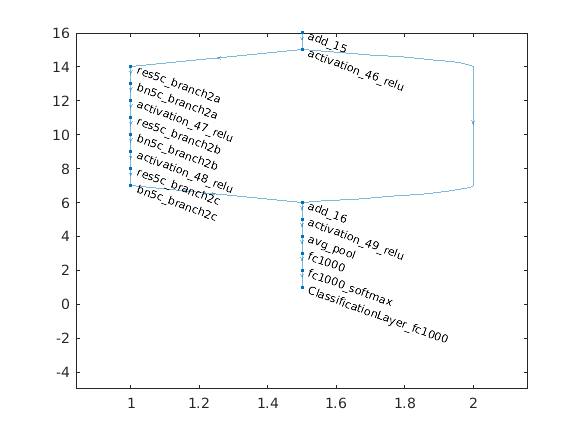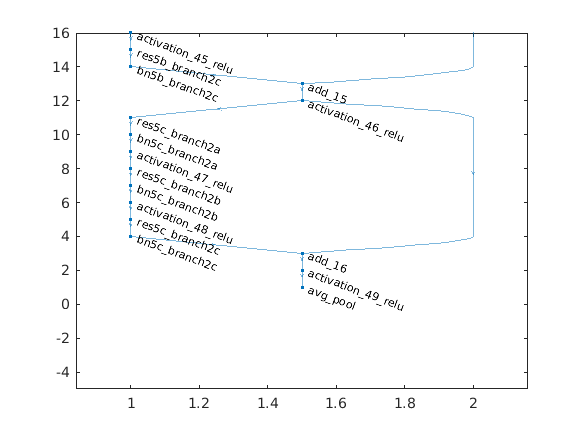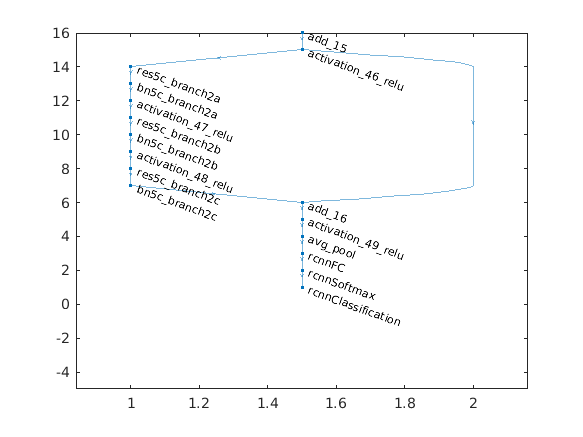# Create R-CNN Object Detection Network

This example shows how to modify a pretrained ResNet-50 network into an R-CNN object detection network. The network created in this example can be trained using `trainRCNNObjectDetector`.

```% Load pretrained ResNet-50. net = resnet50(); % Convert network into a layer graph object to manipulate the layers. lgraph = layerGraph(net);```

The procedure to convert a network into an R-CNN network is the same as the transfer learning workflow for image classification. You replace the last 3 classification layers with new layers that can support the number of object classes you want to detect, plus a background class.

In ResNet-50, the last three layers are named fc1000, fc1000_softmax, and ClassificationLayer_fc1000. Display the network, and zoom in on the section of the network you will modify.

```figure plot(lgraph) ylim([-5 16])``````% Remove the last 3 layers. layersToRemove = { 'fc1000' 'fc1000_softmax' 'ClassificationLayer_fc1000' }; lgraph = removeLayers(lgraph, layersToRemove); % Display the results after removing the layers. figure plot(lgraph) ylim([-5 16])```Add the new classification layers to the network. The layers are setup to classify the number of objects the network should detect plus an additional background class. During detection, the network processes cropped image regions and classifies them as belonging to one of the object classes or background.

```% Specify the number of classes the network should classify. numClassesPlusBackground = 2 + 1; % Define new classfication layers newLayers = [ fullyConnectedLayer(numClassesPlusBackground, 'Name', 'rcnnFC') softmaxLayer('Name', 'rcnnSoftmax') classificationLayer('Name', 'rcnnClassification') ]; % Add new layers lgraph = addLayers(lgraph, newLayers); % Connect the new layers to the network. lgraph = connectLayers(lgraph, 'avg_pool', 'rcnnFC'); % Display the final R-CNN network. This can be trained using trainRCNNObjectDetector. figure plot(lgraph) ylim([-5 16])```